# Dual_EC_DRBG backdoor: a proof of concept

## What’s this ?

Dual_EC_DRBG is an pseudo-random number generator promoted by NIST in NIST SP 800-90A and created by NSA. This algorithm is problematic because it has been made mandatory by the FIPS norm (and should be implemented in every FIPS approved software) and some vendors even promoted this algorithm as first source of randomness in their applications. edit: I’ve been told it’s not the case anymore in FIPS-140-2 but the cat is already out of the bag

If you still believe Dual_EC_DRBG was not backdoored on purpose, please keep reading.
In 2007 already, Dan Shumow and Niels Ferguson from Microsoft showed that Dual_EC_DRBG algorithm could be backdoored. Twitter also uncovered recently that this algorithm was even patented in 2004 by Dan Brown (Not the Da Vinci guy, the Certicom one) as a “key escrow mechanism” (government jargon/lingo for trapdoor/backdoor).
I will go a little bit further in explaining how it works and give a proof-of-concept code, based on OpenSSL FIPS. This is in the best of my knowledge the only public proof of concept published today. (correct me if I’m wrong).

## Dual_EC_DRBG in a nutshell

The PRNG works as following: it takes a seed that goes through a hashing algorithm. This data is then “fed” into two Elliptic Curve points, P and Q, before being slightly transformed and output.

In order to understand how the algorithm (and the backdoor) works, let’s see the relevant maths from Elliptic Curves:

### Elliptic curves

Many types of elliptic curves exist. They are classified in function of their properties and equations. We’re going to see here the curve NIST-p256 which is one of the three curves being used by Dual_EC_DRBG. NIST-p384 and NIST-p521 have very similar characteristics. I’ll try to (poorly) give you the basic theoretics for EC, but I’m no mathematician. Please forgive me and correct me if I’m wrong.

A curve is a set of points that follow a group structure. The curve is defined over several parameters (for NIST GFp curves):

• Equation: all the members of that group (called points) must satisfy this equation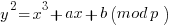• Prime modulus p: a prime number used to define the finite field Z/pZ in which the equation elements are defined.
• The order r: this is the order of the EC and the total number of points into the group.
• a and b: fixed integers used in curve’s equation. a is set to -3 in NIST GF(p) curves.
• A Generator point defined by Gx and Gy: This point is considered as the base element of the group.

Curve members are points. A point is a pair of coordinates X and Y that satisfy the curve’s equation. They are written as capital letters such as G, P or Q. Points have some characteristics from groups:

• They have an addition operation (+) defined between two points. (eg. P + Q).
• This addition is commutative and associative.
• Since you can add a point with itself as many time as you want, it has a scalar multiplication, which is the multiplication of a scalar (0..n) with a point and results in another point.
• That scalar multiplication is associative/commutative (a(bP) = b(aP)).
• This scalar should be in the group of integers modulo r (the order of the curve).

The group of Elliptic Curves is very useful in cryptography, because an equation such as iQ = P is very easy to resolve for Q or P (if you know i) but very hard to resolve for i (if all you know is P and Q). This is the Discrete logarithm problem in the EC group. That’s why most of the time the points are used as public parameters/keys and scalars as private counterparts.

All NIST curves have different parameters p, r, a, b and points G. These parameters have been constructed from a sha1 hash of a public seed but nobody knows how the seed itself has been chosen.

Enough on the theory, let’s study the PRNG !

## Dual_EC_DRBG internals

Dual_EC_DRBG is defined in NIST SP800-90A page 60. It is an algorithm generating an infinite number of pseudo-random sequences from a single seed, taken in the first step or after an explicit reseed.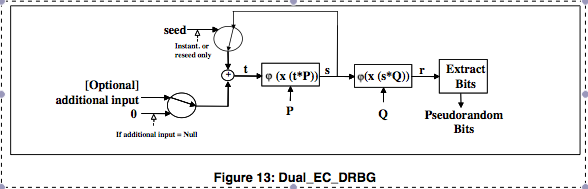It is unfortunate that SP800-90A and the presentation from Microsoft use conflicting terminology (variable names). So I will use these variables: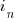: Internal seed value.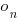: External (output) value.
You can also see in the document two functions: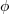and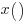.is a function that does a mapping between a large integer and binary data. It doesn’t do much, in fact we can happily ignore it.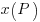simply gets the X coordinate of a point, as the X and Y coordinates are mostly redundant (as we’re going to see later). If we unroll the inductive feedback loop on the first two generated outputs, we get this: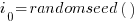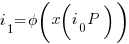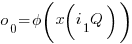output(30 least significant bytes of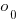)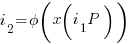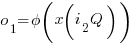output(30 least significant bytes of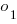)

## An attack

Let’s begin working onand look if we can guessfrom its content. We can see thatis the X coordinate of a point, with 16 bits missing (we lost the 2 most significant bytes in the output process). In a NIST GFp curve, There are for every value of X, zero, one or two points on the curve. So we have at most 17 bits of bruteforce to do to recover the original point A. Let’s work on the hypothesis that we know the point A and it is equal (by definition) to A =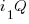.

Then the equation: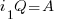but if we have a (secret) relation between P and Q such as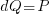with d = secret number (our backdoor !):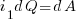multiplicating each side by d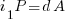(replacing dQ with P)

If you look carefully at the unrolled algorithm, you will notice that if we know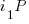we can calculate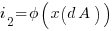and we have all the required information to calculate subsequent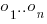and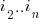. All we need to do is to guess a value of A (based on a bruteforce approach), multiply it by the secret value d, then multiply the resulting scalar with Q, strip two bytes and publish the output. It is also very interesting that if we learn (in a practical attack) the first 32 bytes generated by this PRNG, the 30 first bytes give us candidates for A and the remaining two bytes can be used to validate our findings. If the X value had been output on 32 bytes, we would have an one over two chance of success because of the two coexisting points on same coordinate X. (Remember from high school, second degree equations can have two solutions).

## Generating the constants

As you have seen before, for our backdoor to work we need to choose the P and Q points in order to have the secret key to the backdoor. We have chosen to define P = dQ, however, that can’t work, because P is a generator for the EC group and its value has already been fixed. So, we have to find a value e such as Q = eP. This value can be calculated :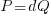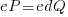(mult. by e)

We need to find a value e such as ed = 1 for the curve C. The equation to resolve is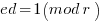where r is the order of the EC.
The value of e is the inverse of d modulo r. We can then use that value to generate Q.

```/* 256 bits value randomly generated */
unsigned char d[]=
"\x75\x91\x67\x64\xbe\x30\xbe\x85\xd1\x50\x09\x19\x50\x8a\xf4\xb5"
"\x7a\xc7\x09\x22\x07\x32\xae\x40\xac\x3e\xd5\xfe\x2e\x12\x25\x2a";
d_bn = BN_new();
assert(d_bn != NULL);

BN_bin2bn(d, 32, d_bn);
/* ensure d is well inside the group order */
EC_GROUP_get_order(curve, r, bn_ctx);
BN_mod(d_bn, d_bn, r, bn_ctx);
/* calculate Q = d * Generator + (NULL * NULL) */
ret = EC_POINT_mul(curve, my_Q, d_bn, NULL, NULL, bn_ctx);
assert(ret == 1);

/* calculate e = d^-1 (mod r) */
e_bn = BN_new();
assert(e_bn != NULL);

/* invert d to get the value of e */
assert(NULL != BN_mod_inverse(e_bn, d_bn, r, bn_ctx));```

(note: I know I mixed up e with d between the code and blog post but that doesn’t change anything at all.)

## Implementation

You can find the proof of concept code on my github. I’ll comment how it works:

### Install OpenSSL/FIPS

Most of the work needed for this POC actually was fighting with OpenSSL FIPS mode (getting it to compile at first) and finding the good APIs to use. OpenSSL FIPS and OpenSSL are two different software that share some codebase. I had to fetch a specific commit of OpenSSL FIPS (one that would compile) and patch it a little to have a few functions from Bignums usable from inside my application. I haven’t been able to mix FIPS and regular libcrypto, because of header incompatibilities (or a bug in my code I though was caused by incompatibilities). The README explains the steps to take (please read it).

### Recover point A

If you remember, we have the 30 least significant bytes of the X coordinate, that means we need to bruteforce our way into A point candidates. This can be easily done in a loop over the 2^16 possibilities. OpenSSL doesn’t provide any way of recovering a point from a single coordinate (there exists a point compression algorithm, but it is so badly patented that it’s not implemented anywhere). We have to resolve the curve’s equation: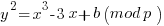Resolving such an equation for y is not so different from the equation resolving you learned at high school:

```for (prefix = 0; prefix <= 0x10000 ; ++prefix){
x_bin = prefix >> 8;
x_bin = prefix & 0xff;
BN_bin2bn(x_bin, 32, x_value);
//bnprint("X value", x_value);

/* try to find y such as */
/* y^2 = x^3 - 3x + b (mod p) */
/* tmp1 = x^2 */
ret = BN_mod_mul(tmp1, x_value, x_value, &curve->field, bn_ctx);
assert(ret == 1);

ret = BN_set_word(tmp2, 3);
assert(ret == 1);

/* tmp1 = x^2 - 3 */
ret = BN_mod_sub(tmp1, tmp1, tmp2, &curve->field, bn_ctx);
assert(ret == 1);

/* tmp1 = (x^2 -3) * x */
ret = BN_mod_mul(tmp1, x_value, tmp1, &curve->field, bn_ctx);
assert(ret == 1);

/* tmp1 = x^3 - 3x + b */
ret = BN_mod_add(tmp1, tmp1, b_bn, &curve->field, bn_ctx);
assert(ret == 1);

//bnprint("Y squared", tmp1);
if (NULL != BN_mod_sqrt(y_value, tmp1, &curve->field, bn_ctx)) {
//printf("value %x match !\n", prefix);
if(verbose)
bnprint("calculated Y", y_value);

BN_mod_sub(y_value, zero_value, y_value, &curve->field, bn_ctx);
if(verbose)
bnprint("calculated Y opposite", y_value);
test_candidate(buffer + 30, x_value, y_value, bn_ctx);
valid_points += 2;
}
}```

I mentioned that for every X, there were zero, one or two solutions: zero if the square root fails (not all elements of Z/pZ are quadratic residues), one if the result is 0 and two for all other answers. There are then two valid points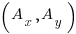and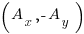where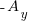is the opposite of the first value modulo p. Explanation (thanks Rod):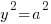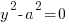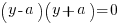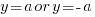### Recover PRNG state and generate next block

This part is pretty straightforward. We import the estimated x and y values, verify that they are in the curve (they should !), then multiply that point with the secret value. We then multiply Q with the resulting scalar and we get 30 bytes of the next output. If the two first bytes match, we have successfully guessed the 28 remaining bytes. That attack can recover everything that’s output by that PRNG till a reseed.

```/* create the point A based on calculated coordinates x and y */
ret = EC_POINT_set_affine_coordinates_GFp(curve, point, x, y, bn_ctx);
assert(ret == 1);
/* Normally the point should be on curve but we never know */
if (!EC_POINT_is_on_curve(curve, point, bn_ctx))
goto end;

/* calculates i2 = phi(x(e.A)) */
ret = EC_POINT_mul(curve, point, NULL, point, e_bn, bn_ctx);
assert(ret ==1);

ret = EC_POINT_get_affine_coordinates_GFp(curve, point, i2x, NULL, bn_ctx);
assert(ret ==1);
if(verbose)
bnprint ("i2_x", i2x);

/* calculate o1 = phi(x(i2 * Q)) */
ret = EC_POINT_mul(curve, point, NULL, my_Q, i2x, bn_ctx);
assert(ret == 1);
ret = EC_POINT_get_affine_coordinates_GFp(curve, point, o1x, NULL, bn_ctx);
if(verbose)
bnprint ("o1_x", o1x);
BN_bn2bin(o1x, o1x_bin);
if (o1x_bin == buffer && o1x_bin == buffer){
printf("Found a match !\n");
bnprint("A_x", x);
bnprint("A_y", y);
print_hex("prediction", o1x_bin + 4, 28);
}```

### Let’s run it !

``` aris@kalix86:~/dualec\$ ./dual_ec_drbg_poc s at start of generate: E9B8FBCFCDC7BCB091D14A41A95AD68966AC18879ECC27519403B34231916485 [omitted: many output from openssl] y coordinate at end of mul: 0663BC78276A258D2F422BE407F881AA51B8D2D82ECE31481DB69DFBC6C4D010 r in generate is: 96E8EBC0D507C39F3B5ED8C96E789CC3E6861E1DDFB9D4170D3D5FF68E242437 Random bits written: 000000000000000000000000000000000000000000000000000000000000 y coordinate at end of mul: 5F49D75753F59EA996774DD75E17D730051F93F6C4EB65951DED75A8FCD5D429 s in generate: C64EAF10729061418EB280CCB288AD9D14707E005655FDD2277FC76EC173125E [omitted: many output from openssl] PRNG output: ebc0d507c39f3b5ed8c96e789cc3e6861e1ddfb9d4170d3d5ff68e242437449e Found a match ! A_x: 96e8ebc0d507c39f3b5ed8c96e789cc3e6861e1ddfb9d4170d3d5ff68e242437 A_y: 0663bc78276a258d2f422be407f881aa51b8d2d82ece31481db69dfbc6c4d010 prediction: a3cbc223507c197ec2598e6cff61cab0d75f89a68ccffcb7097c09d3 Reviewed 65502 valid points (candidates for A) PRNG output: a3cbc223507c197ec2598e6cff61cab0d75f89a68ccffcb7097c09d3 ```
Yikes !

## Conclusion

It is quite obvious in light of the recent revelations from Snowden that this weakness was introduced by purpose by the NSA. It is very elegant and leaks its complete internal state in only 32 bytes of output, which is very impressive knowing it takes 32 bytes of input as a seed. It is obviously complete madness to use the reference implementation from NIST and I’m not the only one to be angry about it. You could use it with custom P and Q values, but that’s very seldom possible. Nevertheless having a whole EC point parameter leaked in the output makes it too easy to distinguish from real random and should never have been made into any specs at all. Let’s all bury that PRNG and the “security” companies bribed by NSA to enable backdoors by default for thirty silver coins.

edits: fixed Dan Brown’s employer name, changed a variable name to avoid confusion, fixed counting error 28 bytes to 30 bytes
note: I did not break the official algorithm. I do not know the secret value used to compute the Q constant, and thus cannot break the default implementation. Only NSA (and people with access to the key) can exploit the PRNG weakness.
note2: Some questions were raised about the security of that secret value. The secret value I speak of is the scalar used to generate Q. As EC is a cyclicgroup, it is proven that for every P and Q there is a value such as eP = Q.
How long would it be to bruteforce that value ? Finding the value e is equivalent to resolving the discrete logarithm problem in NIST-p256. ANSSI tells that GF(p) 256 bits has the same security as a 128bits symmetric cipher encryption key. Keys up to 80 bits are believed to be impossible to crack today, so we can say it’s more likely to be stolen by an NSA employee than being cracked.

edit3: NIST-p256 is a cyclic group and that’s what makes the note2 relation possible
edit4: There’s an awesome video explaining elliptic curves and Dual-ec-drbg.

## 65 Replies to “Dual_Ec_Drbg backdoor: a proof of concept”

1.drakes00 says:

Just for me to understand right, what you’re basically telling is “Knowing d such that Q = dP, we are able to predict the next random bit sequences” ?

For my culture, is there a way to perform the opposite deduction, like, for a given state (and maybe additional information), would we be able to predict former generated bit sequences ?

2.Trey says:

Hey, very interesting read. has this got anything to do with the heartbleed vulnerability in OPENSSL? my uneducated guess tells me that it does

3.Guarana Dong says:

Trey,

This doesn’t have anything to do with the “heart bleed” coined memory leak vulnerability attributed to openssl.

There however is a pattern of similar/closely related vulnerabilities related to openssl/dual_ec_drb vulnerabilities.

Look at the attributes of the dual_ec_drbg vulnerability, and openssl vulnerabilities, and you will see patterns, and preferred vectors of exploitation by the nsa. There are probably a LOT more undetected exploits out there that utilize same methods/behaviors as this article covers/openssl vulnerability, but they haven’t been discovered yet.

4.ویزای سنگاپور says:

﻿
عبد تا بوسیله حال نبا به این نظم در
اینترنت نخوانده بودم بسیار جذاب و
کامل بود و مقاله جالب ایشان درخصوص وافر از ویزیت کنندگان اینترنت
مناسب است

5. ﻿
محتوا ی این پیام از نظر کثرت از افراد
مفت و کامل نیست ولی ازخودگذشته از محتواها سایت ها و بلاگ ها پیرامون این مطلب در وب وجود دارند که می توان از آنها استفاده
بهتری داشت

6.lecalvin.be says:

Deze spellen zijn allemaal gratis te spelen.

my site lecalvin.be

7. ﻿
من این پیام را خواندم و می توانم این یادداشت را به اشخاص دیگر غصه پیشنهاد کنم